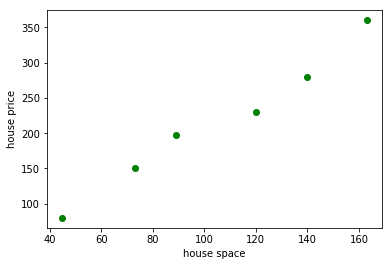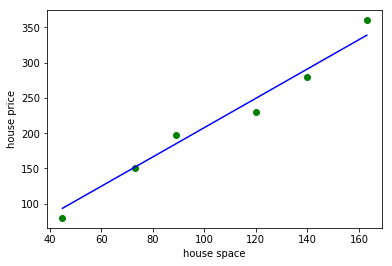02 November 2017

## 相关概念

• 梯度 : 表示某一函数在一点处变化率最快的方向向量(可理解为这点的导数/偏导数)
• 样本 : 实际观测到的数据集，包括输入和输出(本文的样本数量用 m 表述,元素下标 i 表示)
• 特征 : 样本的输入(本文的特征数量用 n 表示,元素下标 j 表示)
• 假设函数 : 用来拟合样本的函数，记为 $h_\theta(X)$ ($\theta$ 为参数向量, X 为特征向量)
• 损失函数 : 用于评估模型拟合的程度，训练的目标是最小化损失函数，记为 $J(\theta)$
• 线性假设函数 :

其中 X 为特征向量, $\theta_j$为模型参数， $x_j$ 是特征向量的第 j 个元素(令$x_0$=1)。

• 经典的平方差损失函数如下：

其中 m 为样本个数， $X_i$ 为样本特征集合的第 i 个元素（是一个向量）， $y_i$ 是样本输出的第i个元素, $h_\theta(X_i)$ 是假设函数。

注意：输入有多个特征时，一个样本特征是一个向量。假设函数的输入是一个特征向量而不是特征向量里面的一个元素

## 梯度下降法

• 根据经验设计假设函数和损失函数，以及假设函数所有 $\theta$ 的初始值
• 对损失函数求所有 $\theta$ 的偏导（梯度）: $\dfrac{\partial J(\theta)}{\partial \theta_j}$
• 使用样本数据更新假设函数的 $\theta$，更新公式为: $\theta_j = \theta_j - \alpha \cdot \dfrac{\partial J}{\partial \theta_j}$

其中 $\alpha$ 为更新步长（调整参数的灵敏度，灵敏度太高容易振荡，灵敏度过低收敛缓慢）

## 例子

%matplotlib inline
import numpy as np
import matplotlib.pyplot as plt

spaces = [45, 73, 89, 120, 140, 163]
prices = [80, 150, 198, 230, 280, 360]
spaces, prices = np.array(spaces), np.array(prices)
plt.scatter(spaces, prices, c='g')
plt.xlabel('house space')
plt.ylabel('house price')
plt.show()

## 显示房屋面积和房屋价格的散点图%matplotlib inline
import numpy as np
import matplotlib.pyplot as plt

## theta 初始值
theta0 = 0
theta1 = 0

## 如果步长选择不对，则 theta 参数更新结果会不对
step = 0.00005

x_i0 = np.ones((len(spaces)))

# 假设函数
def h(x) :
return theta0 + theta1 * x

# 损失函数
def calc_error() :
return np.sum(np.power((h(spaces) - prices),2)) / 6

# 损失函数偏导数( theta 0)
def calc_delta0() :
return step * np.sum((h(spaces) - prices) * x_i0) / 6

# 损失函数偏导数( theta 1)
def calc_delta1() :
return step * np.sum((h(spaces) - prices) * spaces) / 6

# 循环更新 theta 值并计算误差，停止条件为
#  1. 误差小于某个值
#  2. 循环次数控制
k = 0
while True :
delta0 = calc_delta0()
delta1 = calc_delta1()
theta0 = theta0 - delta0
theta1 = theta1 - delta1
error = calc_error()
# print("delta [%f, %f], theta [%f, %f], error %f" % (delta0, delta1, theta0, theta1, error))
k = k + 1
if (k > 10 or error < 200) :
break

print(" h(x) = %f + %f * x" % (theta0, theta1))

# 使用假设函数计算出来的价格，用于画拟合曲线
y_out = h(spaces)

plt.scatter(spaces, prices, c='g')
plt.plot(spaces, y_out, c='b')
plt.xlabel('house space')
plt.ylabel('house price')
plt.show()

# 绿色的点是房屋面积和价格数据
# 蓝色的线是我们使用梯度下降法拟合出来的曲线

 h(x) = 0.016206 + 2.078464 * x$\theta$ 向量更新形式，令

%matplotlib inline
import numpy as np
import matplotlib.pyplot as plt

## 输入数据格式:
##  1. 一个特征的是一维数组，表示样本
##  2. 多个特征的是二维数组，列表示特征数，行表示样本数
spaces = np.array([45, 73, 89, 120, 140, 163])
prices = np.array([80, 150, 198, 230, 280, 360])

# 步长
step = 0.00005

## 先计算输入的特征个数, 然后根据特征数生成 theta，并在样本数据前面插入一列全1数据
def genrate_model(inputs) :
_features = 2
_samples = inputs.shape
if len(inputs.shape) == 2 :
_features = inputs.shape + 1
_x0 = np.ones(_samples)
_theta = np.zeros(_features)
return np.c_[_x0, inputs], _theta, _samples

## 假设函数：输入数据矩阵与theta向量向乘, 返回多项式结果的一维矩阵
def h_a(x) :
return (theta * x).sum(axis=1)

## 损失函数
def e_a(x,y) :
return np.sum(np.power((h_a(x) - y),2)) / m

## delta函数：计算偏导乘以补偿
def delta_a(x, y) :
return step * ((h_a(x) - y) * np.transpose(x)).sum(axis=1) / m

## 系统的特征数 + 1
x_data, theta, m = genrate_model(spaces)
y_data = prices

## 重新计算 delta 并更新 theta
k = 0
while True:
_d = delta_a(x_data, y_data)
theta = theta - _d
error = e_a(x_data, y_data)
# print("delta", _d, "theta ", theta , ", error ", error, "k ", k)
k = k + 1
if (k > 10 or error < 200) :
break;

# 打印 theta 结果，可以看出与上面 python 代码计算的结果是一致的。
print("theta array : " , theta)

theta array :  [ 0.01620597  2.07846445]


## 注意点

• 对于凸函数来说 $\theta$ 的初始值多少关系不大，对于非凸函数的初始值选择不当会陷入局部最优解
• 梯度下降的步长取决于样本数据，根据实际运行效果进行调整
• 误差最小值也是跟数据相关的，需要根据实际情况给定结束条件
• 对于多个特征值的系统，需要使用 z-score 方法对数据进行归一化,公式如下:

## 分类

• 批量梯度下降法（BGD）：使用所有样本进行计算，慢但准确度好
• 随机梯度下降法（SGD)：每次使用1个样本进行计算,快但准确度欠缺
• 小批量梯度下降法：每次使用a个样本进行计算，是BGD和SGD的折中方案